A curve is governed by the equation y=sinx, then what is the area enclosed by the curve and x-axis between x =0 and x =$\mathrm{\pi }$ is (shaded region)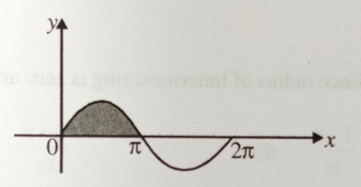1. 1 units

2. 2 units

3. 3 units

4. 4 units

Concept Questions :-

Integration
High Yielding Test Series + Question Bank - NEET 2020

Difficulty Level:

Calculate the area of disk of radius 'a' using integration

1. $\frac{{\mathrm{\pi a}}^{2}}{2}$

2. ${\mathrm{\pi a}}^{2}$

3. $\frac{3}{2{\mathrm{\pi a}}^{2}}$

4. $2{\mathrm{\pi a}}^{2}$

Concept Questions :-

Integration
High Yielding Test Series + Question Bank - NEET 2020

Difficulty Level:

The acceleration of a particle starting from rest varies with time according to relation, . Find the velocity of the particle at time instant t.

1. $\alpha {t}^{2}+\beta t$

2. $\alpha {t}^{2}+\frac{\beta t}{2}$

3. $\frac{\alpha {t}^{2}}{2}+\beta t$

4. $2\alpha {t}^{2}+\beta t$

Concept Questions :-

Integration
High Yielding Test Series + Question Bank - NEET 2020

Difficulty Level:

The displacement of particle is zero at t=0 and at t=t it is x. It starts moving in the x direction with velocity, which varies as $v=k\sqrt{x}$, where k is constant. The velocity-

1. varies with time

2. is independent to time

3. inversely proportional to time

4. inversely proportional to acceleration

Concept Questions :-

Integration
High Yielding Test Series + Question Bank - NEET 2020

Difficulty Level:

The acceleration of a particle is given as $a=3{x}^{2}$. At t=0, v=0, x=0, the velocity at t =2 sec will be-

1.  0.05 m/s

2. 0.5 m/s

3. 5 m/s

4. 50 m/s

Concept Questions :-

Integration
High Yielding Test Series + Question Bank - NEET 2020

Difficulty Level:

The acceleration of a particle is given by a=3t and at t=0, v=0, x=0. The velocity and displacement at t = 2 sec will be-

1. 6 m/s, 4 m

2. 4 m/s, 6 m

3. 3 m/s, 2 m

4. 2 m/s, 3 m

Concept Questions :-

Integration
High Yielding Test Series + Question Bank - NEET 2020

Difficulty Level:

The 9 kg block is moving to the right with a velocity of 0.6 m/s on a horizontal surface when a force F, whose time variation is shown in the graph, is applied to it at time t = 0. Calculate the velocity v of the block when t= 0.4s. The coefficient of kinetic fricton is ${\mu }_{k}=0.3$[ This question is only for Dropper and XII batch]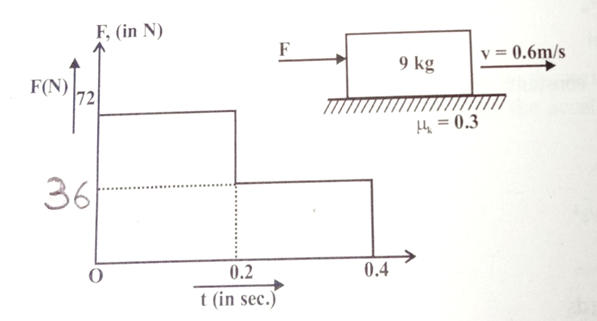1. 0.6 m/s

2. 1.2 m/s

3. 1.8 m/s

4. 2.4 m/s

Concept Questions :-

Integration
High Yielding Test Series + Question Bank - NEET 2020

Difficulty Level:

A 10 kg mass moves along x-axis. Its acceleration as a function of its position is shown in the figure. What is the total work done on the mass by the force as the mass moves from x=0 to x=8 cm [This question is only for Dropper and XII batch]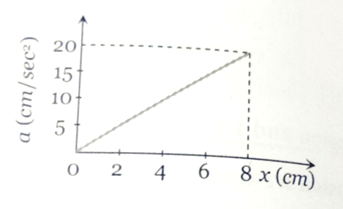1.

2.

3.

4.

Concept Questions :-

Integration
High Yielding Test Series + Question Bank - NEET 2020

Difficulty Level:

The relationship between force and position is shown in the figure given (in one dimensional case). Find the work done by the force in displaying a body from x= 1 cm to x= 5cm is   [This question is only for Dropper and XII batch]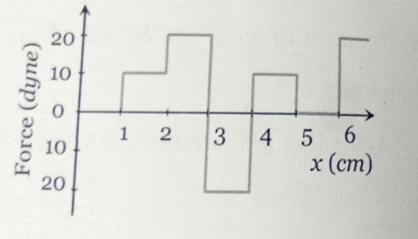1. 10 erg

2. 20 erg

3. 30 erg

4. 40 erg

Concept Questions :-

Integration
High Yielding Test Series + Question Bank - NEET 2020

Difficulty Level:

The graph between the resistive force F acting on a body and the distance covered by the body is shown in the figure. The mass of the body is 25 kg and initial velocity is 2 m/s. When the distance covered by the body is 4 m, its kinetic energy would be    [This question is only for Dropper and XII batch]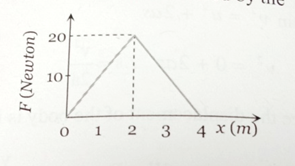1. 50 J

2. 40 J

3. 20 J

4. 10 J

Concept Questions :-

Integration